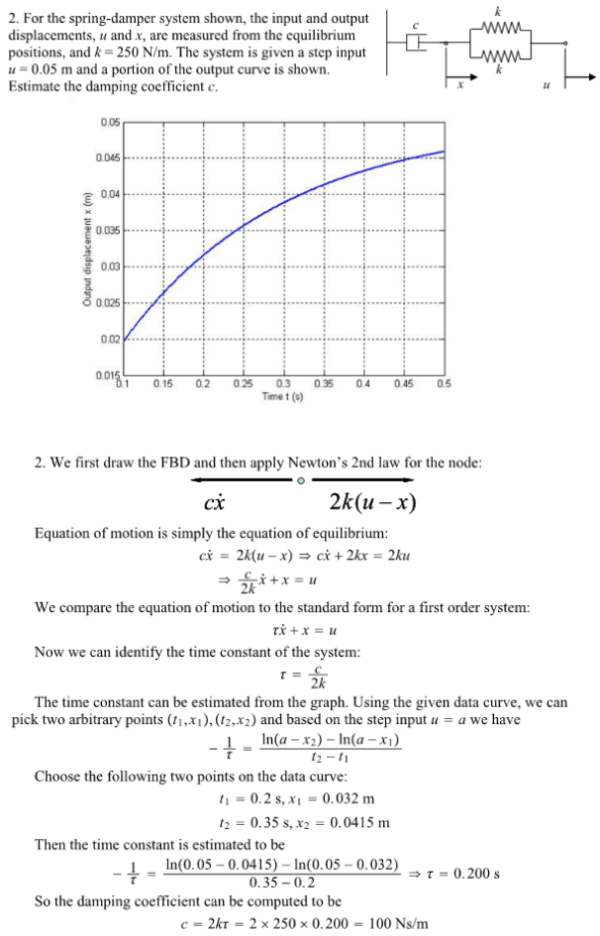For the spring-damper system shown, the input and output displacemetns, u and x, are measured from the equilibrium positions, and k=250 N/m. The system is given a step input u=0.05 m and a portion of the output curve is shown. Estimate the damping coefficient c.For the spring-damper system shown, the input and output displacemetns, u and x, are measured from the equilibrium positions, and k=250 N/m. The system is given a step input u=0.05 m and a portion of the output curve is shown. Estimate the damping coefficient c.

System Dynamics Page 2 dynamics dynamics dynamics dynamics dynamics dynamics dynamics System dynamics Page 3